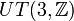# Unitriangular matrix group:UT(3,Z)

View a complete list of particular groups (this is a very huge list!)[SHOW MORE]

## Definition

### As a reduced free group

Abstractly, this group is a free class two group on a generating set of size two. Hence, it is a reduced free group.

### As a matrix group

This group, denoted$UT(3,\mathbb{Z})$ or$U(3,\mathbb{Z})$, is defined as the unitriangular matrix group of degree three over the ring of integers. Explicitly, it is the group, under multiplication:$\left \{ \begin{pmatrix} 1 & a_{12} & a_{13} \\ 0 & 1 & a_{23} \\ 0 & 0 & 1 \\\end{pmatrix} \mid a_{12},a_{13},a_{23} \in \mathbb{Z} \right \}$

The group is also sometimes called the integral Heisenberg group.

### Definition by presentation

The group can be defined by means of the following presentation:$\langle x,y,z \mid [x,y] = z, xz = zx, yz = zy \rangle$

We can relate this with the matrix group definition by setting:$x = \begin{pmatrix} 1 & 1 & 0 \\ 0 & 1 & 0 \\ 0 & 0 & 1 \\\end{pmatrix}, \qquad y = \begin{pmatrix} 1 & 0 & 0 \\ 0 & 1 & 1 \\ 0 & 0 & 1 \\\end{pmatrix}, \qquad z = \begin{pmatrix} 1 & 0 & 1 \\ 0 & 1 & 0 \\ 0 & 0 & 1 \\\end{pmatrix}$

### Structures

The group has the structure of an arithmetic group.

## Arithmetic functions

Function Value Similar groups Explanation
nilpotency class 2 The derived subgroup and center are both equal to the subgroup$\left \{ \begin{pmatrix} 1 & 0 & a_{13} \\ 0 & 1 & 0 \\ 0 & 0 & 1 \\\end{pmatrix} \mid a_{13} \in \mathbb{Z} \right \}$
derived length 2 Follows from nilpotency class being 2.
Frattini length 2 The Frattini subgroup also coincides with the derived subgroup and center, and it is isomorphic to the group of integers, which is a Frattini-free group.
Hirsch length 3 We can use a polycyclic series that starts with the center, then goes to the subgroup$\left \{ \begin{pmatrix} 1 & a_{12} & a_{13} \\ 0 & 1 & 0 \\ 0 & 0 & 1 \\\end{pmatrix} \mid a_{12}, a_{13} \in \mathbb{Z} \right \}$, and then goes to the whole group. Each of the quotient groups is isomorphic to$\mathbb{Z}$.
polycyclic breadth 3 We can use a polycyclic series that starts with the center, then goes to the subgroup$\left \{ \begin{pmatrix} 1 & a_{12} & a_{13} \\ 0 & 1 & 0 \\ 0 & 0 & 1 \\\end{pmatrix} \mid a_{12}, a_{13} \in \mathbb{Z} \right \}$, and then goes to the whole group. Each of the quotient groups is isomorphic to$\mathbb{Z}$.

## Group properties

Property Satisfied? Explanation
abelian group No
nilpotent group Yes
group of nilpotency class two Yes
metacyclic group No
polycyclic group Yes
metabelian group Yes
supersolvable group Yes